## University Question Bank

Unit 1

Part A

April May - 2010

1. Determine the energy of the discrete time sequence x(n) = { 1, 2+j2,2,2-2j}

2. Check whether the system characterized by the equation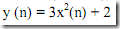is linear or not.

May June - 2011

4. State sampling theorem

Nov Dec – 2011

5. Define causal theorem

6. State sampling theorem

May June 2012

7. Define aliasing and Nyquist rate

8. Test the system y (n) = n2x (n) for the time invariance

May June 2013

9. What is power signal?

Part B

April May – 2010

1. Explain the following with respect to discrete-time system:

(i) Causality

(ii) Linearity

(iii) Stability

(iv) Shift-invariant

2. Explain what is quantization?

3. Explain sampling theorem for band pass signals.

4. Determine the Nyquist sampling rate for the following signal:

5. Explain about analog-to-digital conversion

May June – 2011

6. Check the linearity, time invariance, causality and stability for the systems described by

7. State and prove sampling theorem

Nov Dec – 2011

8. Determine which of the following are periodic signals and hence find their period. x1(n) = exp(-0.1 ∏ n)

x2(n) = sin(n ∏ / 4) + sin(n ∏ / 7)

x3(n) = exp(j0.2 ∏n + ∏/2)

x4(n) = U(n) + U(n - 2) - U(n - 4)

9. State and prove Low Pass Sampling Theorem with neat spectra.

May June 2012

10. Explain how sampling can be done with an impulse function. Draw the spectrum of the sampled signal and explain aliasing.

11. Explain the process of reconstruction of the signal from its samples. Obtain the impulse response of an ideal reconstruction filter.

12. Verify and explain whether the following impulse responses describe causal, stable or LTI systems.

May June 2013

13. Explain the time invariant property of systems and find if the system y[n) = x(n)+x(n -1) is time invariant, linear and causal.

14. State the stability property of LTI systems and check if the system defined by y(n) = x(n) + nx(n +1) is stable, linear and causal.

15. Explain the Nyquist sampling theorem and the effect on the signal at different sampling rates.

16. Explain the process of quantization during analog to digital conversion and errors associated with it.

Unit 2

Part A

April May - 2010

1. State the initial value theorem of z-transform

2. Define discrete Fourier series representation of a periodic sequence

May June - 2011

Nov Dec – 2011

5. Define stability of a discrete time system in 2-domain

6. Define transfer function

May June 2012

7. What is the relation between fourier transform and Z transform?

8. What is Dirchlet’s condition?

May June 2013

9. What is the inverse Z transform of 1?

10. Mention two properties of ROC of Z transform.

Part B

April May – 2010

1. Explain the properties of ROC of Z transform

2. Using Z transform find the impulse response of the system described by the difference equation: y(n)-(3/4)y(n-1)+(1/8)y(n-2) = x(n)

3. Find the frequency response of the system with difference equation y(n) + (1/2)y(n-1) = x(n) – x(n-1)

4. ) Find the linear convolution of x(n) = {1,-3,5,-7,9} with h(n) = {8,10,12,14}.

May June – 2011

5. Find the Z-transform of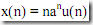and also write the ROC.

6. Find the inverse Z transform of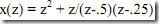for the ROC Z>0.5

7. Find y(n0 if y(n)-(3/4)y(n-1)+(1/8)y(n-2) = (0.25)n for n>0. The initial conditions are y(-1) = 4 and Y(-2) = 10.

Nov Dec – 2011

8. State the Convolution Property of Z Transform and prove the same.

9. Find the inverse Z Transform of X{Z) = ZI(Z – 1)2

10. A discrete time LTI system y(n - 2) + 0.8y(n -1) + 0,15y(n - 2) = 2x(n). Determine (a) The System function (b) Impulse response (c) Frequency response (d) Whether the system is causal and/or stable.

May June 2012

11. using the residue method, find the Inverse Z Transform of x(z) = 1/(z-0.25)(z-0.5), ROC:Z>0.5

12. Determine the Fourier Transform of the signal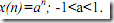May June 2013

13. Find the Z transform of the signal x(n) = (l / 3)n-1 u(n -1).

14. Find the poles of the system and determine if the system is stable.

Unit 3

Part A

April May – 2010

1. Find the 4-point DFT of x(n) = {1,-1,0,1}

2. What is meant by “inplace-computation” in FFT algorithm?

May June – 2011

3. Distinguish circular shifting and shifting in DFT.

4. Compare the number of complex multiplications required for direct calculation and

FFT evolution of N – point DFT if N = 1024.

Nov Dec – 2011

5. What is meant by bit reversed indexing?

6. Determine the number of multiplication required in finding 64-point DFT using

May June 2012

7. What is bin spacing?

8. Compute the DFT of the sequence x(n) = (-1)n for the period N = 16

May June 2013

9. What is meant by radix 2 FFT?

10. Define circular convolution

Part B

April May – 2010

1. Derive the decimation-in-frequency radix-2 FFT algorithm for evaluating DFT of the discrete-time sequence and draw flow graph for 8-point DFT computation.

2. Using FFT algorithm find the inverse DFT of

May June – 2011

3. Find the 8 point DFT of the following sequence using DIT (decimation in time) FFT algorithm

x(n) a {1.-1,-1,-14,1.1,-1}.

4. State and prove the circular frequency shift and circular time shift properties of DFT.

5. Explain the calculation of inverse DFT using FFT algorithm.

Nov Dec – 2011

6. A discrete time LTI system y(n - 2) + 0.8y(n -1) + 0,15y(n - 2) = 2x(n). Determine (a) The System function (b) Impulse response (c) Frequency response (d) Whether the system is causal and/or stable.

7. State and prove any four properties of DFT

8. Find 8 point DFT of given sequence x(n) = {1,2,3,4} using radix-2 DIT-FFT

May June 2012

9. State and prove circular convolution

10. Determine the DFT (8-point) for a continuous time signal, x(t)=sin(2∏ft) with f=50Hz.

11. Determine the response of LTI system by radix-2 FFT. The input sequence is given by x(n) = {-1, 1, 2, 1, -1} and impulse response is h (n) = {-1, 1,-1,1}

May June 2013

12. Explain the important features of Decimation in frequency FFT algorithm.

13. Obtain the DFT of x(n) = {1, 2, 3, 4, 4, 3, 2, 1} using Decimation in time FFT algorithm.

14. Compute the N point DFT of x{n) = an u(n) for cases |a| < 1 and |a| = 1.

15. State and prove the circular time shift property of DFT

Unit 4

Part A

April May – 2010

1. What are the properties of FIR filters?

2. What is bilinear transformation?

May June – 2011

3. What is the main objective of impulse invariant transformation?

4. What is meant by frequency warping?

Nov Dec – 2011

5. Define linear phase shift filter

6. Mention the advantage of Bilinear Transformation.

May June 2012

7. What is frequency warping?

8. Define phase delay and group delay

May June 2013

9. What is the advantage of bilinear transformation?

10. Write the general form of difference equation of a FIR filter.

Part B

April May – 2010

1. Explain the desirable characteristics of the window function.

2. Draw the Cascade realization using minimum number of multipliers for the system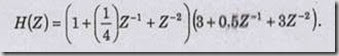3. Design a low pass FIR filter of order 7 with cut off frequency ∏/3 rad/sec using Hanning window

4. Design a digital Chebyshev filter to meet the constraints :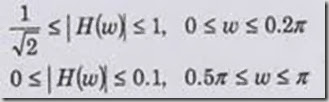by using bilinear transformation with sampling Period T = 1 sec.

May June – 2011

5. Design and realize a digital low pass filter using the bilinear transforms method to satisfy the following characteristics:

• Monotonic stop band and pass band
• 3.01 dB cutoff frequency of 0.5 ∏ rad
• Magnitude down at least 15 dB at 0.75∏r rad.

6. Obtain a parallel realization for the following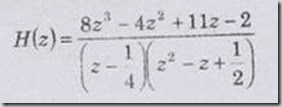Use direct form II realization for each section

7. Explain the different windows used in FIR filter design.

Nov Dec – 2011

8. Design a FIR Filter for the following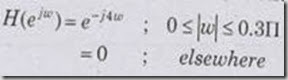in the band 0 to ∏. Use hamming window.

9. Convert the following analog filter into digital using IIM method.

H(S) = IS2 /(S2 + 0.3S+0.02)

10. Compare Butterworth and Chebyshev approximations

May June 2012

11. Design and realize a low pass filter using a rectangular window by taking 9 samples of w(n) and with a cutoff frequency of 1.2 rad/sec.

12. Design a Butterworth low pass digital filter satisfying the following conditions using bilinear transformation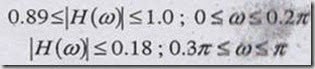May June 2013

13. Explain the properties of Chebychev filters.

14. Find the order N and the transfer function of analog Chebychev low pass filter for the following specification: Pass band ripple 3 dB and pass band cut off frequency 1 KHz, stop band attenuation of 16 dB at stop band frequency of 2 KHz.

15. Mention the characteristic features of FIR filters

16. Design a FIR filter with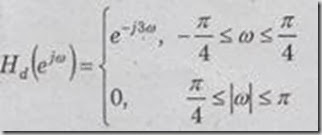Using Hanning window and N = 7

Unit 5

Part A

April May – 2010

1. What is pipelining?

2. What is meant by limit cycle oscillations?

May June – 2011

3. Define limit cycle

4. Write about the accumulators in TMS 320C54X.

Nov Dec – 2011

5. What is the advantage of Harvard architecture?

6. Define Dead Band

May June 2012

7. What is Dead band of Filter?

8. What is meant by limit cycles?

May June 2013

9. What is product quantization error?

10. What is pipeline depth and what is its value of the TMS320C54X?

Part B

April May – 2010

1. Draw the black diagram of Harvard architecture and explain.

2. Write short notes on :(i) Direct addressing (ii) Memory-mapped register addressing.

3. Explain the different buses and their functions of TMS320C54X processor.

4. Explain quantization effects in FIR filter.

May June – 2011

5. Explain the TMS 320C54X internal hardware with a neat diagram.

6. Explain the errors introduced by quantization with necessary expression

7. Illustrate the impact of quantization of filter coefficients on the poles and zeros with an example.

Nov Dec – 2011

8. Draw the architecture outlines of TMS320C54X processors and explain its features.

9. Write short note on (a) Truncation and rounding (b) Coefficient Quantization.

May June 2012

10. With a neat block diagram explain the architecture of TMS320C54X

11. Study the limit cycle behavior of the system descr ibed by w(n)=Q[aw(n—l)]+x(n) where w(n) is the output of the filter and Q[.] is quantization. Assume that a = 7/8, x(0)=3/4 and x(n)= 0 for n> 0.

May June 2013

12. Describe the architecture of TMS320C54X and explain the functions of the control arithmetic logic unit, parallel arithmetic logic unit and various registers in it.

13. Explain zero input limit cycle oscillations.

14. Explain the output noise due to A/D conversion of the input x (n).